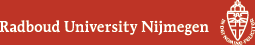## Geometry Seminar - Abstracts

### Talk

Thursday 23 February 2017, 16:00 - 17:00 in HG03.085
Harm Derksen (University of Michigan, Ann Arbor)
Matrix Invariants

### Abstract

The determinant of an n x n matrix is an invariant polynomial of degree n that is invariant under left and right multiplication with matrices in SL(n). It generates in the sense that every other invariant polynomial is a polynomial expression in the determinant. In this talk we consider the simultaneous left and right action of SL(n) on m-tuples of n x n matrices. I will explain a joint result with Visu Makam that shows that invariants of degree at most n^6 are sufficient to generate all polynomial invariants. These results have applications in Algebraic Complexity Theory.

(Back to geometry seminar schedule)